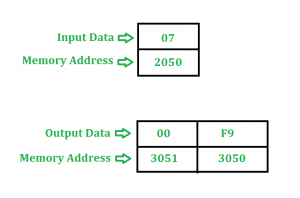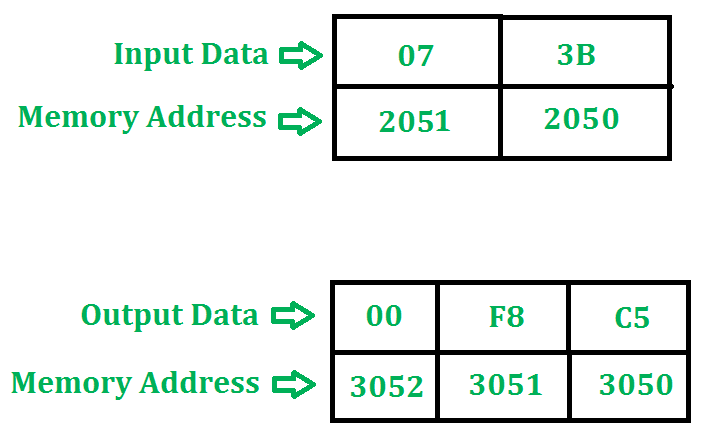GeeksforGeeks App
Open AppBrowser
Continue

# 8085 programs to find 2’s complement with carry | Set 2

Problem-1: Find 2’s complement of an 8 bit number stored at address 2050. Result is stored at address 3050 and 3051. Starting address of program is taken as 2000.

Example –Algorithm –

1. We are taking complement of the number using CMA instruction.
2. Then adding 01 to the result.
3. The carry generated while adding 01 is stored at 3051.

Program –

Explanation – Registers used: A, H, L

1. LDA 2050 loads content of 2050 in A
2. CMA complements the contents of A
3. INR A increases A by 01
4. MOV L, A copies contents of A in L
5. MVI A 00 moves 00 in A
6. ADC A adds A, A, Carry and assigns it to A
7. MOV H, A copies contents of A in H
8. SHLD 3050 stores value of H at memory location 3051 and L at 3050
9. HLT stops executing the program and halts any further execution

Problem-2: Find 2’s complement of a 16 bit number stored at address 2050 and 2051. Result is stored at address 3050, 3051 and 3052. Starting address of program is taken as 2000.

Example –Algorithm –

1. We are taking complement of the numbers using CMA instruction.
2. Then adding 0001 to the result using INX instruction.
3. The carry generated while adding 0001 is stored at 3052.

Program –

Explanation – Registers used: A, H, L

1. LHLD 2050 loads content of 2051 in H and content of 2050 in L
2. MOV A, L copies contents of L in A
3. CMA complements contents of A
4. MOV L, A copies contents of A in L
5. MOV A, H copies contents of H in A
6. CMA complements contents of A
7. MOV H, A copies contents of A in H
8. INX H adds 0001 in HL
9. MVI A 00 moves 00 in A
10. ADC A adds A, A, Carry and stores result in A
11. SHLD 3050 stores value of H at memory location 3051 and L at 3050
12. STA 3052 stores value of A at memory location 3052
13. HLT stops executing the program and halts any further execution

• It is a simple and efficient method to find the 2’s complement of a binary number using the carry.

• It is faster than the traditional method of finding the 2’s complement by inverting all bits and adding 1.

• It requires fewer instructions and registers compared to other methods.